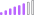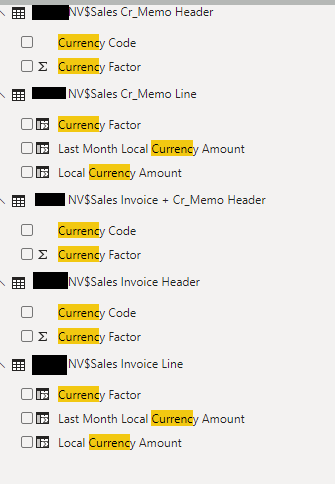cancel
Showing results for
Did you mean:Post Patron

## How to get newly added columns into appended table?

Hello,

I have a table sales and a table credit memo. THey both contain the same lines. I added additional columns that calculated the currency conversion. They also contain the same name.

I appended these 2 tables and everything works fine, except for the added the columns. They do not appear in the new appended table.As you can see Invoice Line and Credit Memo Line contain 3 new tables. I appended the Invoice line and Credit Memo Line and these  3 tables didn't appear in the new table. How can I append those as well?

2 ACCEPTED SOLUTIONSSuper User

@Niels_T , if you added a column in DAX they will not append in Power Query. Power Query is the data preparation layer before the DAX model layer.

If you can create those formulas in the power query, they will be part of append.Super User

@Niels_T , Lookup need few steps

refer

https://exceloffthegrid.com/power-query-lookup-values-using-merge/

https://eriksvensen.wordpress.com/2019/02/28/powerquery-replicate-doing-an-excel-vlookup-in-m/

power query have if then else (case sensitive)

Local Currency Amount = if 'xxxxNV\$Sales Invoice Line'[Currency Factor] = 0 then 'xxxxNV\$Sales Invoice Line'[Amount] else 'xxxxNV\$Sales Invoice Line'[Amount] / 'xxxxNV\$Sales Invoice Line'[Currency Factor])

Margin = if 'xxxxNV\$Sales Invoice Line'[Currency Factor] = 0 then 'xxxxNV\$Sales Invoice Line'[Amount] else 'xxxxNV\$Sales Invoice Line'[Amount] / 'xxxxNV\$Sales Invoice Line'[Currency Factor]) - ([Unit Cost (LCY)]*[Quantity (Base)])

Margin % = if 'xxxxNV\$Sales Invoice Line'[Currency Factor] = 0 then 'xxxxNV\$Sales Invoice Line'[Amount] else 'xxxxNV\$Sales Invoice Line'[Amount] / 'xxxxNV\$Sales Invoice Line'[Currency Factor]) - ([Unit Cost (LCY)]*[Quantity (Base)]) / if 'xxxxNV\$Sales Invoice Line'[Currency Factor] = 0 then 'xxxxNV\$Sales Invoice Line'[Amount] else 'xxxxNV\$Sales Invoice Line'[Amount] / 'xxxxNV\$Sales Invoice Line'[Currency Factor])*100

3 REPLIES 3Super User

@Niels_T , if you added a column in DAX they will not append in Power Query. Power Query is the data preparation layer before the DAX model layer.

If you can create those formulas in the power query, they will be part of append.Post Patron

I notice that I cannot use DAX in Power Query. Is it even possible to write these functions in Power Querty then?:

Currency Factor = LOOKUPVALUE('xxxxNV\$Sales Invoice Header'[Currency Factor],'xxxxNV\$Sales Invoice Header'[No_], 'xxxxNV\$Sales Invoice Line'[Document No_])

Local Currency Amount = IF('xxxxNV\$Sales Invoice Line'[Currency Factor] = 0, 'xxxxNV\$Sales Invoice Line'[Amount], 'xxxxNV\$Sales Invoice Line'[Amount] / 'xxxxNV\$Sales Invoice Line'[Currency Factor])

Margin = IF('xxxxNV\$Sales Invoice Line'[Currency Factor] = 0, 'xxxxNV\$Sales Invoice Line'[Amount], 'xxxxNV\$Sales Invoice Line'[Amount] / 'xxxxNV\$Sales Invoice Line'[Currency Factor]) - ([Unit Cost (LCY)]*[Quantity (Base)])

Margin % = IF('xxxxNV\$Sales Invoice Line'[Currency Factor] = 0, 'xxxxNV\$Sales Invoice Line'[Amount], 'xxxxNV\$Sales Invoice Line'[Amount] / 'xxxxNV\$Sales Invoice Line'[Currency Factor]) - ([Unit Cost (LCY)]*[Quantity (Base)]) / IF('xxxxNV\$Sales Invoice Line'[Currency Factor] = 0, 'xxxxNV\$Sales Invoice Line'[Amount], 'xxxxNV\$Sales Invoice Line'[Amount] / 'xxxxNV\$Sales Invoice Line'[Currency Factor])*100Super User

@Niels_T , Lookup need few steps

refer

https://exceloffthegrid.com/power-query-lookup-values-using-merge/

https://eriksvensen.wordpress.com/2019/02/28/powerquery-replicate-doing-an-excel-vlookup-in-m/

power query have if then else (case sensitive)

Local Currency Amount = if 'xxxxNV\$Sales Invoice Line'[Currency Factor] = 0 then 'xxxxNV\$Sales Invoice Line'[Amount] else 'xxxxNV\$Sales Invoice Line'[Amount] / 'xxxxNV\$Sales Invoice Line'[Currency Factor])

Margin = if 'xxxxNV\$Sales Invoice Line'[Currency Factor] = 0 then 'xxxxNV\$Sales Invoice Line'[Amount] else 'xxxxNV\$Sales Invoice Line'[Amount] / 'xxxxNV\$Sales Invoice Line'[Currency Factor]) - ([Unit Cost (LCY)]*[Quantity (Base)])

Margin % = if 'xxxxNV\$Sales Invoice Line'[Currency Factor] = 0 then 'xxxxNV\$Sales Invoice Line'[Amount] else 'xxxxNV\$Sales Invoice Line'[Amount] / 'xxxxNV\$Sales Invoice Line'[Currency Factor]) - ([Unit Cost (LCY)]*[Quantity (Base)]) / if 'xxxxNV\$Sales Invoice Line'[Currency Factor] = 0 then 'xxxxNV\$Sales Invoice Line'[Amount] else 'xxxxNV\$Sales Invoice Line'[Amount] / 'xxxxNV\$Sales Invoice Line'[Currency Factor])*100